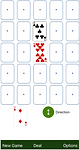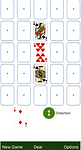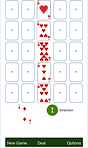top of page

Scores

• Scores are based on 15s, pairs, runs, and flushes.

• Scoring is as follows:

Pair = 2 points

15 = 2 points

Flush = 5 points

Run = 1 point / card

(min of 3 cards in run)

• Scores are counted based on the direction of the player (up/down or left/right).

• Runs, pairs, 15s, and flushes are all counted independently, and the same cards in a row/column can be used to create any combintation thereof.

• Each row or column is counted individually and then all the rows or columns are added up to create the player's final score for the hand.

15s

• 15s are scored when 2 or more cards in the same row or column can be added together to create 15 (i.e. 9 + 6 or 2 + 3 + 10)

• The values for the cards are:

Ace = 1

2 = 2

3 = 3

4 = 4

5 = 5

6 = 6

7 = 7

8 = 8

9 = 9

10 = 10

Jack = 10

Queen = 10

King = 10

• Each possible combination of cards is counted as a distinct 15. For example, a 7-7-8 combintation is counted as two 15s, since two 7-8 combinations are possiblePairs

• Pairs are when two of the same type of card are in the same row or column• Each possible combination of cards is counted as a distinct pair. For example, 3 cards of the same type would be 6 points, since 3 distinct combinations of pairs can be created.

Runs

• Runs are 3 or more consecutive cards in the same row or column

• Order of cards are:

Ace

2

3

4

5

6

7

8

9

10

Jack

Queen

King

• Each possible combination of cards is counted as a distinct run. For example, 5-6-6-7 would be counted as two runs since the 5,7 cards can be coupled with each six independently to create two runs.Flush

• Flushes are when an entire row or column contains cards that are all the same suit.

• They do not need to be in consecutive orderbottom of page